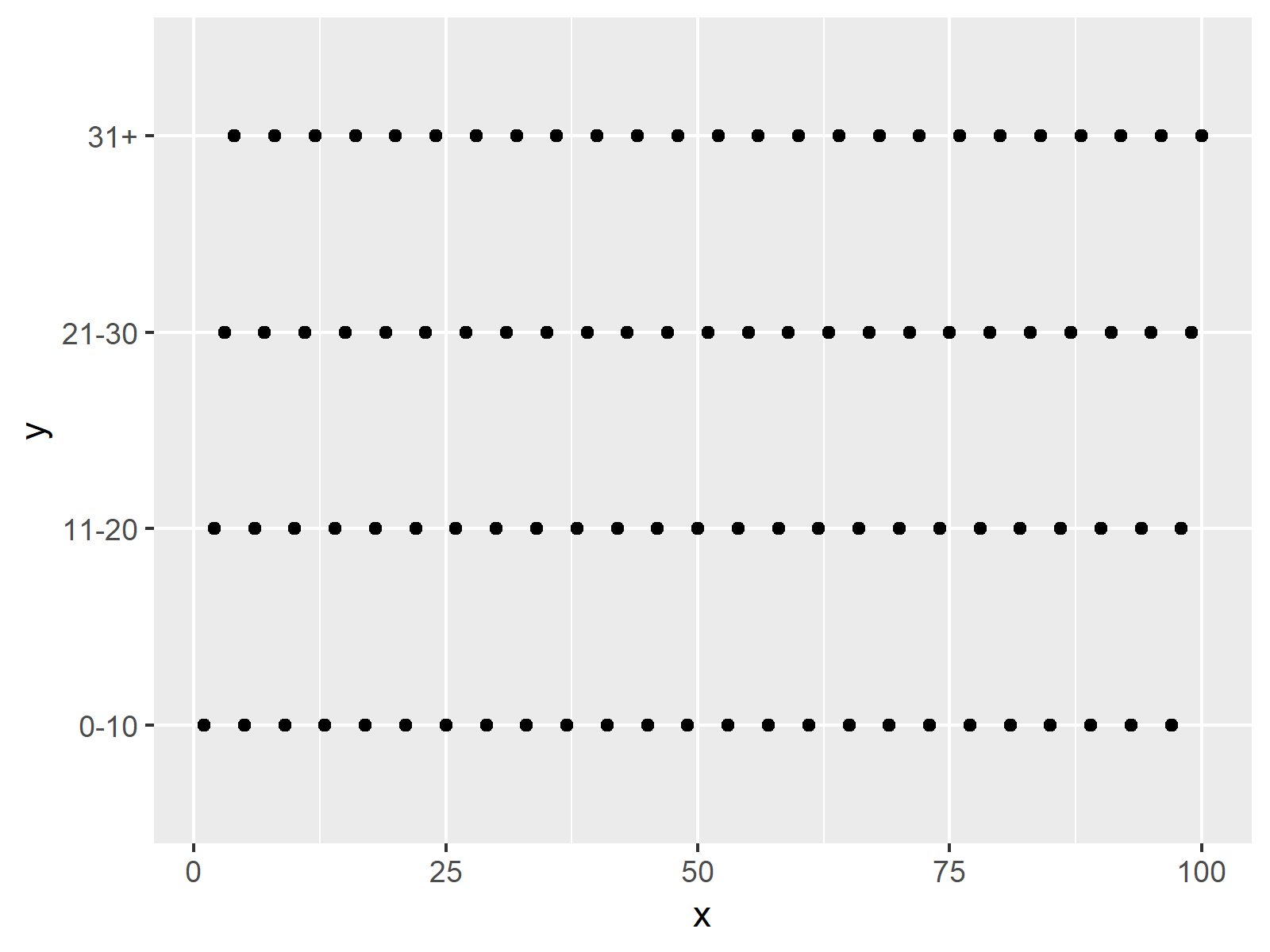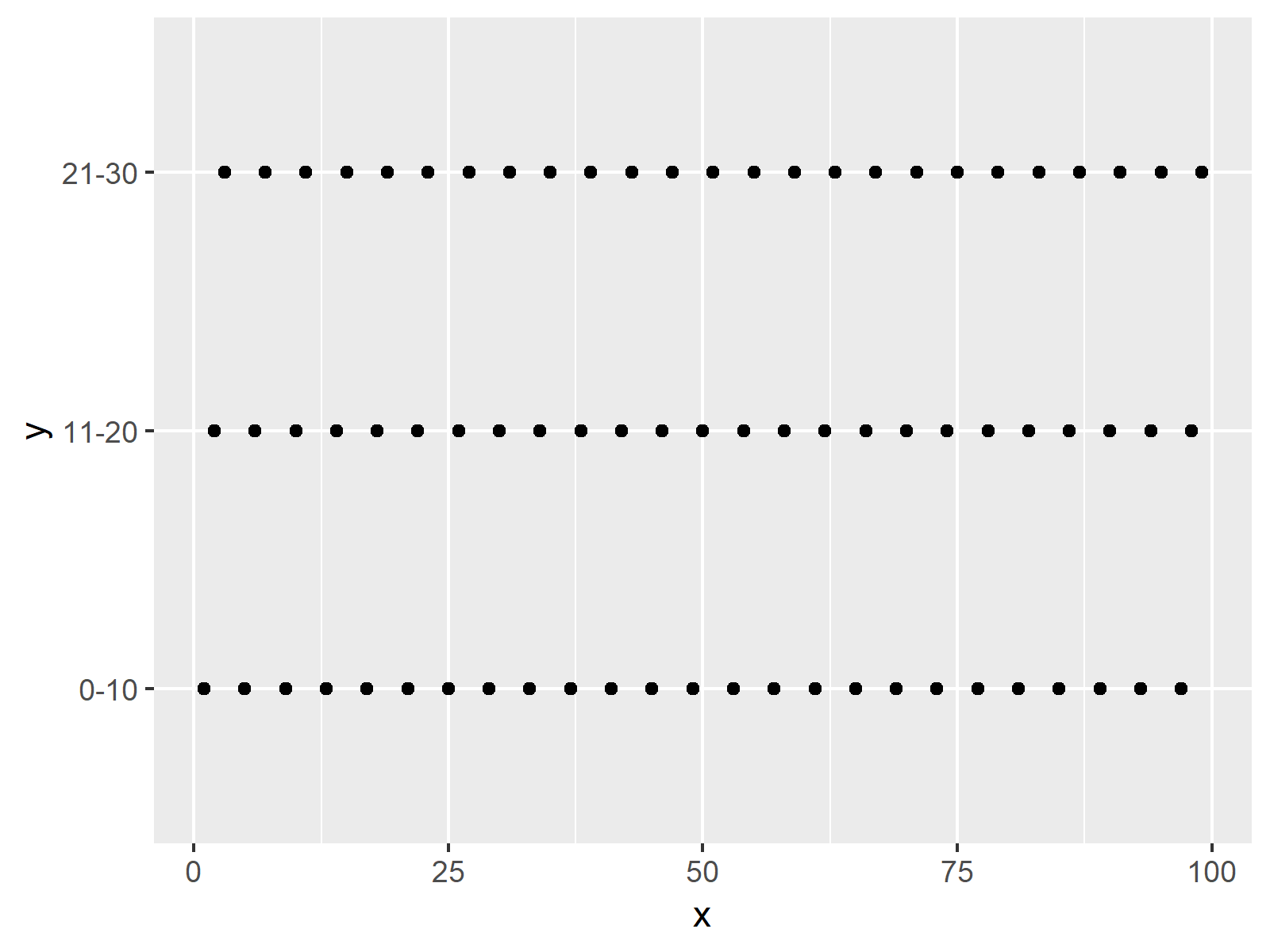# R ggplot2 Error: Discrete Value Supplied to Continuous Scale (2 Examples)

This article explains how to handle the ggplot2 error “Discrete value supplied to continuous scale” in the R programming language.

Let’s get started.

## Example Data, Add-On Packages & Default Graph

The following data is used as basement for this R programming tutorial:

```data <- data.frame(x = 1:100,          # Create example data
y = c("0-10", "11-20", "21-30", "31+"))
#   x     y
# 1 1  0-10
# 2 2 11-20
# 3 3 21-30
# 4 4   31+
# 5 5  0-10
# 6 6 11-20```

Have a look at the previously shown output of the RStudio console. It shows that our example data has two columns. The variable x contains numeric values and the variable y is a factor consisting of four different categories.

If we want to plot our example data with the ggplot2 package, we also have to install and load ggplot2:

```install.packages("ggplot2")            # Install & load ggplot2 package
library("ggplot2")```

Now, we can draw our data as shown below:

```ggplot(data, aes(x, y)) +              # Drawing ggplot2 scatterplot
geom_point()```As shown in Figure 1, we created a ggplot2 graphic showing our example data with the previous R programming code.

Everything worked fine so far, however, this is going to change soon!

## Example 1: Reproduce the Error: Discrete value supplied to continuous scale

In this example, I’ll illustrate how to reproduce the error message “Discrete value supplied to continuous scale” of the ggplot2 package.

Let’s assume that we want to set an axis limit for our plot manually. Then, we might try to use the scale_y_continuous function:

```ggplot(data, aes(x, y)) +              # Trying to set limits on y-axis
geom_point() +
scale_y_continuous(limits = c(0, 30))
# Error: Discrete value supplied to continuous scale```

The RStudio console returns the error message “Error: Discrete value supplied to continuous scale”. The reason for this is that we have tried to limit our axis by numeric values, even though our data is categorical.

Let’s solve this problem!

## Example 2: Fix the Error: Discrete value supplied to continuous scale

In this example, I’ll show how to get rid of the error message “Error: Discrete value supplied to continuous scale”.

As explained before, this error message typically occurs when we want to set an axis limit in a ggplot2 graph.

However, when dealing with factorial data, we can simply create a subset of our input data frame before drawing our plot:

`data_new <- data[data\$y != "31+", ]    # Subsetting data frame`

Now, let’s run the functions of the ggplot2 package again, but this time with the previously created subset of our data frame:

```ggplot(data_new, aes(x, y)) +          # Draw ggplot2 plot of subset
geom_point()```As shown in Figure 2, we plotted a ggplot2 graph showing only a selected part of our data frame with the previous code.

## Video, Further Resources & Summary

If you need more info on the R programming codes of this tutorial, you may want to watch the following video of my YouTube channel. I’m explaining the R programming code of the present article in the video:

Furthermore, you might want to read the other tutorials on statisticsglobe.com.

In summary: In this article, I explained how to deal with “Discrete value supplied to continuous scale” in the R programming language. Let me know in the comments, in case you have further comments and/or questions.

Subscribe to the Statistics Globe Newsletter